## acm第一周练习题

2021/1/28 23:51:34 文章标签:

### 目录

• A - 查成绩
• 思路
• D - 回文数猜想
• 思路
• F - 买鸡问题
• 思路
• H - 最小新整数
• 思路
• I - 赶时间
• 思路
• J - 暖气坏了
• 思路
• K - 完美立方
• 思路
• M - 母牛的故事
• 思路
• 思路2
• N - 无限的路
• 思路
• 思路2
• O - 统计元音
• 思路
• P - 求数列的和
• 思路
• 思路2
• Q - 数字游戏
• 思路
• R - 轻重搭配
• 思路
• 以下是没做出来的题
• B - 放暑假
• C - 展开字符串
• E - 宇航员
• G - 水贴
• 思路
• L - N对数的排列问题
• S - Powered Addition
• T - Puzzle From the Future

13/20

# A - 查成绩

Sample Input
insert zhangsan 90
insert lisi 78
insert xiaoming 86
find xiaoming
find Jack
end
Sample Output
86
-1

## 思路

f=[]
name=[]
s=[]
i=input().split()
while i=='insert':
name.append(i)
s.append(i)
i=input().split()
while i=='find':
f.append(i)
i = input().split()
if i=='end':
break
for i in range(0,len(f)):
q=0
for j in range(0,len(name)):
if f[i]==name[j]:
print(s[j])
q=1
break
if q==0:
print("-1")


# D - 回文数猜想

Input

Output

Sample Input
27228
37649
Sample Output
3
27228—>109500—>115401—>219912
2
37649—>132322—>355553

## 思路

#include <stdio.h>
int researve(int n);
int main()
{
int n,r;
while(scanf("%d",&n)!=EOF)
{
r=n;
int m=0,q=0;
while(m=researve(n),m!= n)
{
n=n+m;
q++;
}
n=r;
printf("%d\n",q);				//记录次数
while(m=researve(n),m!= n)		//回文数即原数与翻转数相等
{
printf("%d--->",n);
n=n+m;                       //把n更新为 m + n;
}
printf("%d\n",n);
}
return 0;
}

int researve(int n) //翻转函数
{
int t=0;
while(n>0)
{
t=t*10+n%10;
n=n/10;
}
return t;
}


# F - 买鸡问题

Sample Input
100
Sample Output
0 25 75
4 18 78
8 11 81
12 4 84

## 思路

x+y+z=n
5x+3y+1/3z=n

n=int(input())
x=-1
f=0
y=(n-(7*x))/4
while x<n:
x=1+x
y=(n-(7*x))//4
z=n-x-y
if x<0 or y<0 or z<0:
continue
if 7*x+4*y==n:
print("%d %d %d" %(x,y,z))
f=1
continue
if f==0:
print("No Answer.")


# H - 最小新整数

t行，每行一个数字，表示从n中删除k位后得到的最小整数。
Sample Input
2
9128456 2
1444 3
Sample Output
12456
1

## 思路

#include <stdio.h>
#include <string.h>
int main()
{
char n;
int t,b,len,flag=1;
scanf("%d",&t);
while(t--){
scanf("%s %d",n,&b);
len=strlen(n);
for(int i=0;i<b;i++){
for(int j=0;j<len-1;j++){
if(n[j]>n[j+1]){
for(int k=j;k<len-1;k++){
n[k]=n[k+1];
}
break;
}
}
len--;
}
for(int i=0;i<len;i++){
printf("%c",n[i]);
}
printf("\n");
}
}


# I - 赶时间

1<h<24,0 m<60,0<s<60。

Sample Input
11:10:10
10:30:30
Sample Output
2380

## 思路

%*c会跳过：

#include<stdio.h>
int main()
{
int h1,m1,s1,h2,m2,s2,time;
scanf("%d%*c%d%*c%d",&h1,&m1,&s1);
scanf("%d%*c%d%*c%d",&h2,&m2,&s2);
if (m1<m2)
{
m1+=60;
h1-=1;
}
if (s1<s2)
{
s1+=60;
m1-=1;
}
h1-=h2;
m1-=m2;
s1-=s2;
time=h1*3600+m1*60+s1;
printf("%d",time);
return 0;
}


# J - 暖气坏了

Sample Input
4 3
1 2 4 8
Sample Output
9

## 思路

#include<stdio.h>
int main()
{
int n,m,i,s,sum=0;
scanf("%d %d",&n,&m);
for (i=0;i<n;i++)
{
scanf("%d",&s[i]);
}
for (i=1;i<n;i++)
{
if (s[i]-s[i-1]<=m)	sum+=s[i]-s[i-1];//小于感冒天数一直感冒
if (s[i]-s[i-1]>m)	sum+=m;//大于感冒天数只感冒m天
}
sum+=m;//最后一次暖气坏的时候感冒的天数
printf("%d",sum);
}


# K - 完美立方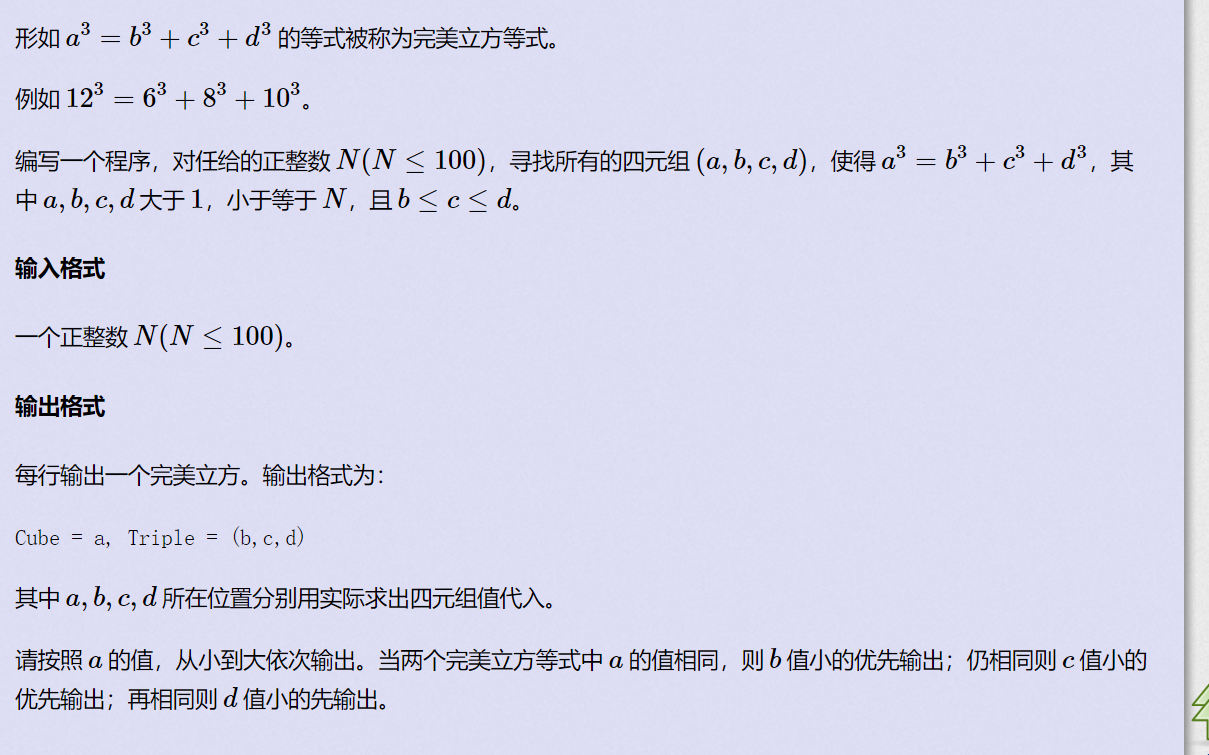Sample Input
24
Sample Output
Cube = 6, Triple = (3,4,5)
Cube = 12, Triple = (6,8,10)
Cube = 18, Triple = (2,12,16)
Cube = 18, Triple = (9,12,15)
Cube = 19, Triple = (3,10,18)
Cube = 20, Triple = (7,14,17)
Cube = 24, Triple = (12,16,20)

## 思路

#include<stdio.h>
int f(int a)
{
return a*a*a;
}
int main()
{
int a=6,b=3,c=4,d=5,n;
scanf("%d",&n);
while(a<=n)
{
if (f(a)==f(b)+f(c)+f(d)&&b<=c&&c<=d)
printf("Cube = %d, Triple = (%d,%d,%d)\n",a,b,c,d);
d++;
if (d>n){
d=2;
c++;
}
if (c>n){
c=2;
b++;
}
if (b>n){
b=2;
a++;
}
}
return 0;
}


# M - 母牛的故事

Sample Input 2
5
Sample Output 2
6

## 思路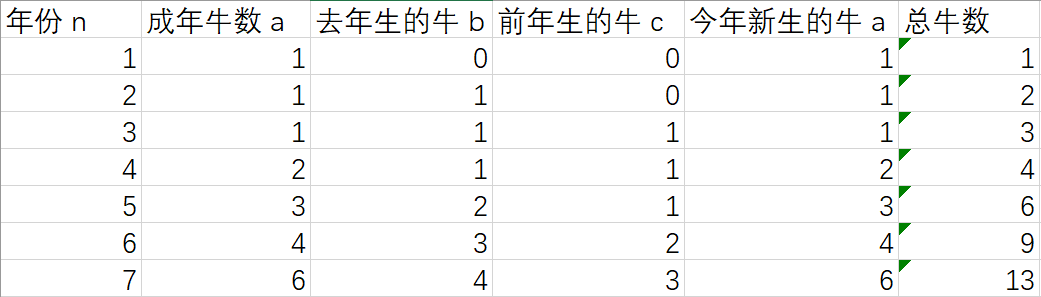#include<stdio.h>
int main()
{
int n,a,b,c;
scanf("%d",&n);
a=1;a=1;a=1;b=0;c=0;c=0;
for (int i=0;i<55;i++)
{
if (i>0) b[i]=a[i-1];
if (i>1) c[i]=b[i-1];
if (i>2) a[i]=c[i-1]+a[i-1];
}
printf("%d",a[n-1]+b[n-1]+c[n-1]);
//printf ("%d\n",a[n-1]);
//printf ("%d\n",b[n-1]);
//printf ("%d\n",c[n-1]);
return 0;
}


## 思路2

#include<stdio.h>
int main(void)
{
int f,i,n;
while(scanf("%d",&n)!=EOF)
{
f=1;f=2;f=3;
for(i=4; i<=n;i++){
f[i]=f[i-3]+f[i-1];
}
printf("%d\n",f[n]);
}
return 0;
}


# N - 无限的路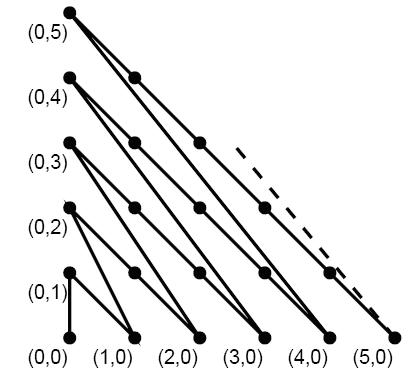Input

Output

Sample Input
5
0 0 0 1
0 0 1 0
2 3 3 1
99 99 9 9
5 5 5 5
Sample Output
1.000
2.414
10.646
54985.047
0.000

## 思路

1.这个老是过不去，不知道为什么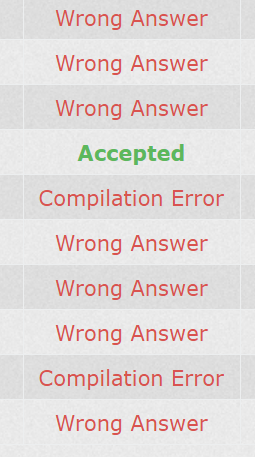#include<stdio.h>
#include<math.h>
double f(double n)
{
return sqrt((n*n)+(n+1)*(n+1));
}
int main()
{
int y1,x2,y2,n,t;
scanf("%d",&n);
double x1,s;
while(n--)
{
scanf("%lf %d %d %d",&x1,&y1,&x2,&y2);
s=0;
if (x1+y1>x2+y2)
{
t=x2;x2=x1;x1=t;
t=y2;y2=y1;y1=t;
}
if (x1+y1<x2+y2)	s+=(x2+y1)*sqrt(2);

if (x1+y1==0)
{
s+=1;
x1=0;y1=1;
}
if (x1+y1==x2+y2)
{
printf("%.3lf\n",s);
continue;
}
x1=x1+y1;y1=0;
y2=x2+y2;x2=0;
while (x1+y1!=x2+y2)
{
if (x1==0)
{
s+=y1*sqrt(2);
x1=y1;
y1=0;

}
if (y1==0)
{
s+=f(x1);
y1=x1+1;
x1=0;
}
}
printf("%.3lf\n",s);
}
return 0;
}


## 思路2

#include <stdio.h>
#include <stdlib.h>
#include<math.h>
int main()
{
int i,x1,x2,y1,y2,s;
double m,n,f;
scanf("%d",&s);
f=0;f=1;
for(i=2;i<=200;i++)
{
f[i]=f[i-1]+sqrt(2*(i-1)*(i-1))+sqrt(i*i+(i-1)*(i-1));
}
while(s--)
{
scanf("%d%d%d%d",&x1,&y1,&x2,&y2);
m=f[x1+y1]+sqrt(2*x1*x1);
n=f[x2+y2]+sqrt(2*x2*x2);
printf("%.3lf\n",fabs(m-n));
}
return 0;
}


# O - 统计元音

Input

Output

a:num1
e:num2
i:num3
o:num4
u:num5

Sample Input
2
aeiou
my name is ignatius
Sample Output
a:1
e:1
i:1
o:1
u:1

a:2
e:1
i:3
o:0
u:1

## 思路

#include<string.h>
int main()
{
char s;
int n;
scanf("%d",&n);
getchar();
int a1[n],e1[n],i1[n],o1[n],u1[n];
while(n--)
{
int a=0,e=0,i=0,o=0,u=0;
gets(s);
int t,len;
len=strlen(s);
for(t=0;t<len;t++)
{
if (s[t]=='a')
a++;
if (s[t]=='e')
e++;
if (s[t]=='i')
i++;
if (s[t]=='o')
o++;
if (s[t]=='u')
u++;
}
printf("a:%d\ne:%d\ni:%d\no:%d\nu:%d",a,e,i,o,u);
if (n!=0) printf("\n\n");
else printf("\n");
}
return 0;
}


# P - 求数列的和

Input

Output

Sample Input
81 4
2 2
Sample Output
94.73
3.41

## 思路

#include<stdio.h>
#include<math.h>
double array(int n,int m)
{
double k=n,i,sum;
sum=k;
for(i=2;i<=m;i++)
{
k=sqrt(k);
sum+=k;
}
return sum;
}
int main()
{
int m,n;
while(scanf("%d%d",&n,&m)!= EOF)
{
printf("%.2lf\n",array(n,m));
}
return 0;
}


## 思路2

#include <stdio.h>
#include <math.h>
int main(void)
{
int n, m;
double sum, a;
while(scanf("%d%d", &n, &m) != EOF)
{
sum = n;
a = n;
while(--m) {
a = sqrt(a);
sum += a;
}
printf("%.2f\n", sum);
}
return 0;
}


# Q - 数字游戏

Sample Input
00010100
Sample Output
2
Sample Explain

## 思路

#include<stdio.h>
int main()
{
char s;
gets(s);
int a=0;
for (int i=0;i<8;i++)
{
if (s[i]=='1')	a++;
}
printf("%d",a);
return 0;
}


# R - 轻重搭配

n个同学去动物园参观，原本每人都需要买一张门票，但售票处推出了一个优惠活动，一个体重为x的人可以和体重至少为2x配对，这样两人只需买一张票。现在给出了n个人的体重，请你计算他们最少需要买几张门票?

1和9配对，7和3配对，剩下5,5单独,一共买四张票。
Sample Input
6
1 9 7 3 5 5
Sample Output
4

## 思路

int main()
{
int n,a,i=0,ct,en;
scanf("%d",&n);
for(;i<n;i++) scanf("%d",&a[i]);
Sort(a,n-1,0,n-1);
ct=n/2;en=n;
for(i=0;i<n/2;i++)
{
while(2*a[i]>a[ct]&&ct<n) ct++;
if (ct>=n) break;
en--;
ct++;
}
printf("%d\n",en);
}


void swap(int *a, int *b)
{
int temp;
temp = *a;
*a = *b;
*b = temp;
}
void Sort(int *arr, int maxlen, int begin, int end)
{
int i, j;
if (begin < end) {
i = begin + 1;
j = end;
while (i < j) {
if(arr[i] > arr[begin]) {
swap(&arr[i], &arr[j]);
j--;
} else {
i++;
}
}
if (arr[i] >= arr[begin]) {
i--;
}
swap(&arr[begin], &arr[i]);
Sort(arr, maxlen, begin, i);
Sort(arr, maxlen, j, end);
}
}


# B - 放暑假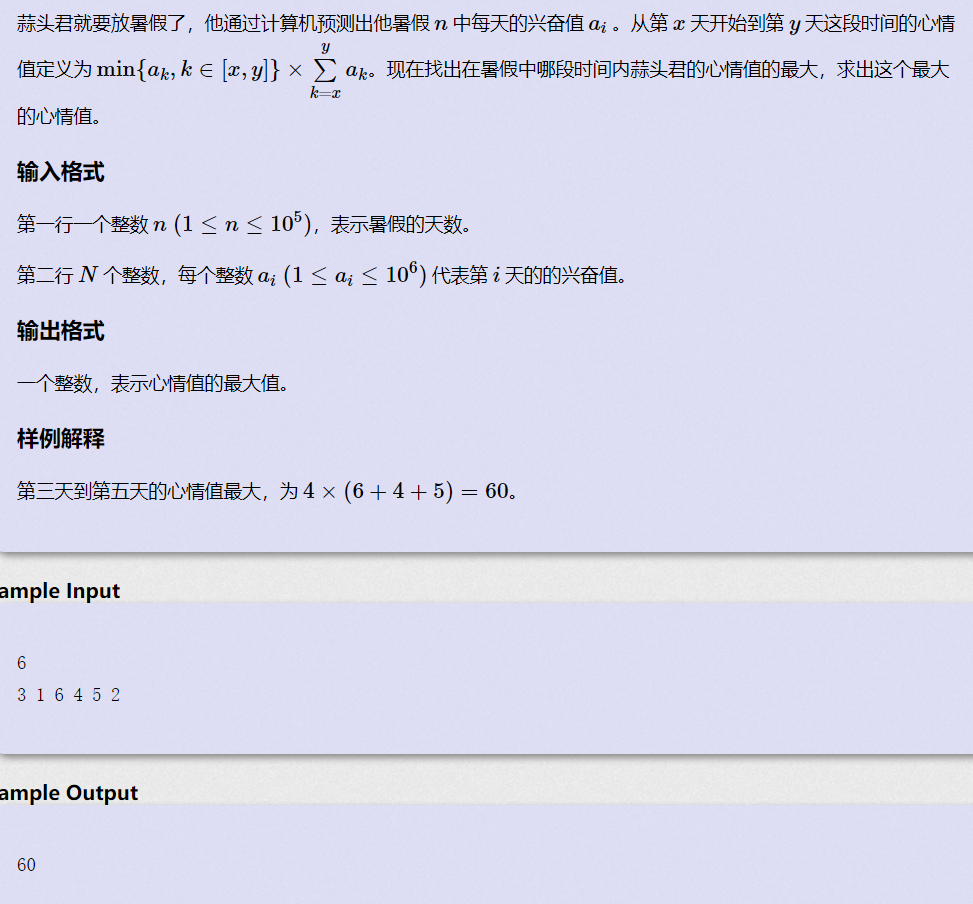# C - 展开字符串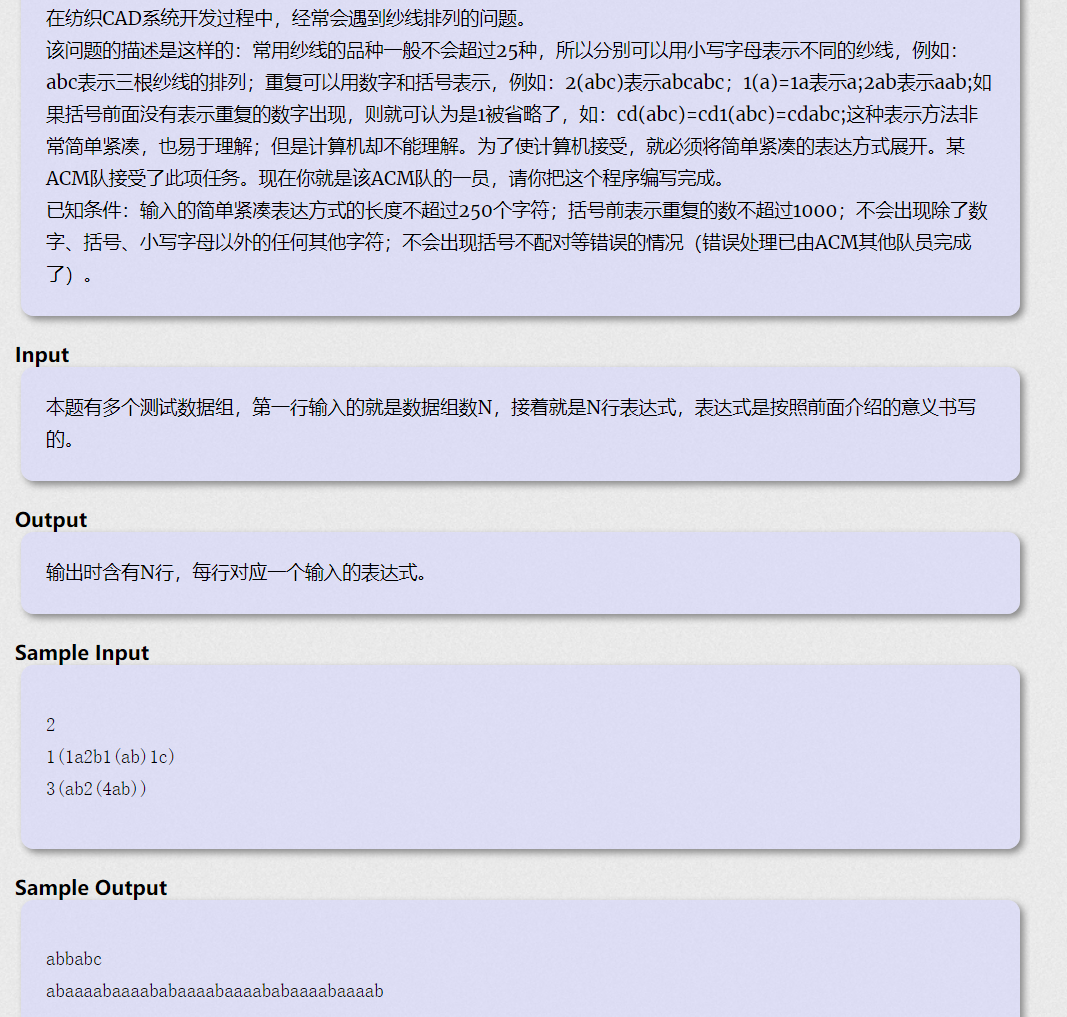# E - 宇航员

宇航员在太空中迷失了方向，在他的起始位置现在建立一个虚拟xyz坐标系，称为绝对坐标系，宇航员正面的方向为x轴正方向，头顶方向为z轴正方向，则宇航员的初始状态如下图所示：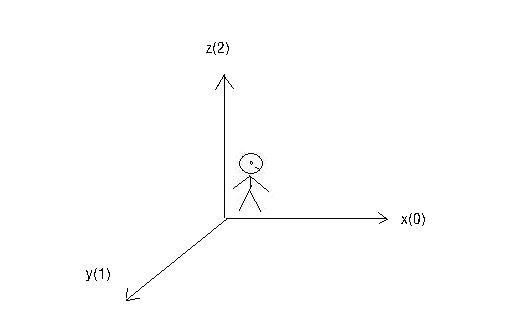请根据宇航员对自己在相对方向上移动的描述确定宇航员最终的绝对坐标和面向的绝对方向。对在相对方向上移动的描述及意义如下：
forward x 　向前走x米。
back x　先转向后，再走x米。
left x 先转向左，再走x米。
right x 先转向右，再走x米。
up x 先面向上，再走x米。
down x 先面向下，再走x米。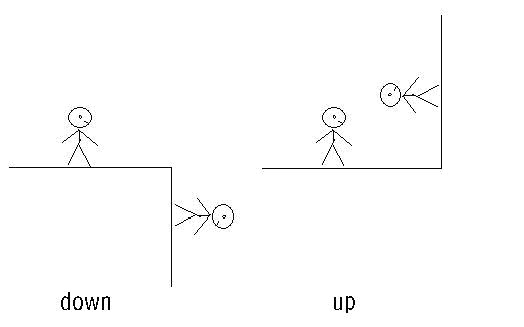Input

Output

Sample Input
1
6
left 10
right 11
up 12
down 13
forward 14
back 15
Sample Output
23 -10 12 3

# G - 水贴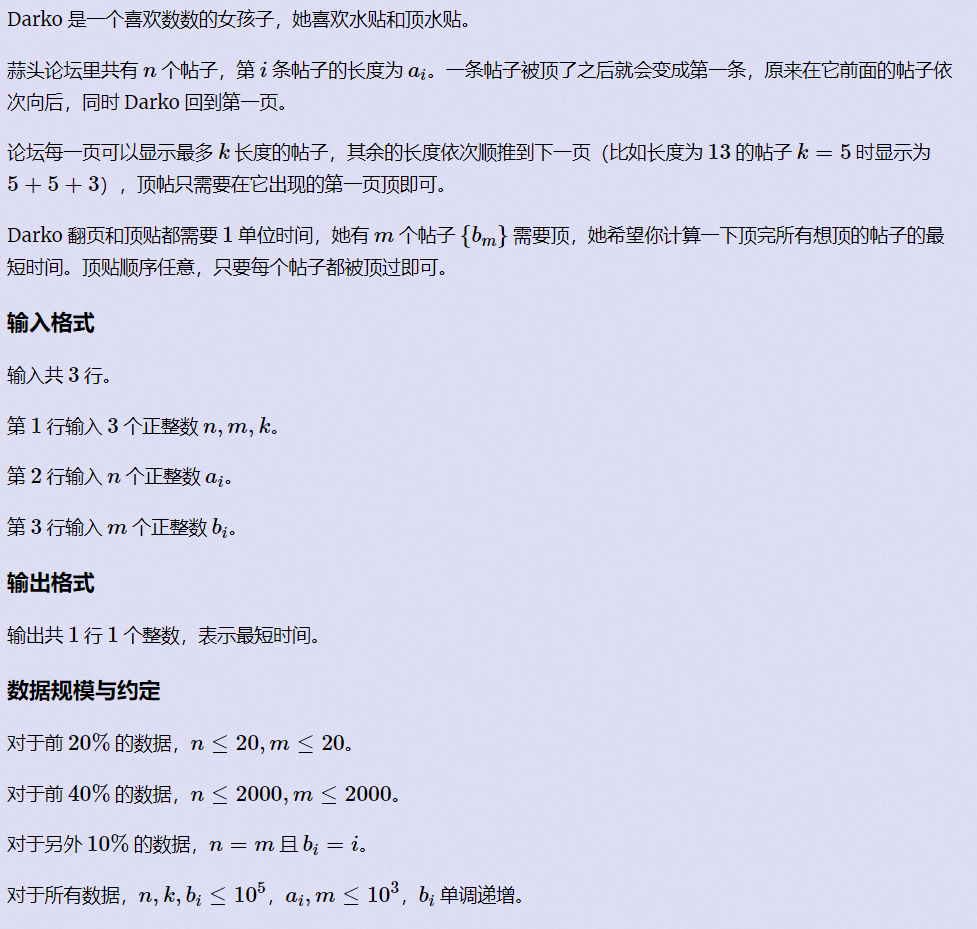Sample Input
5 3 5
4 5 3 4 3
2 4 5
Sample Output
8

## 思路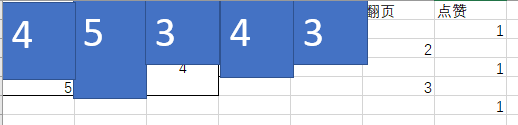# L - N对数的排列问题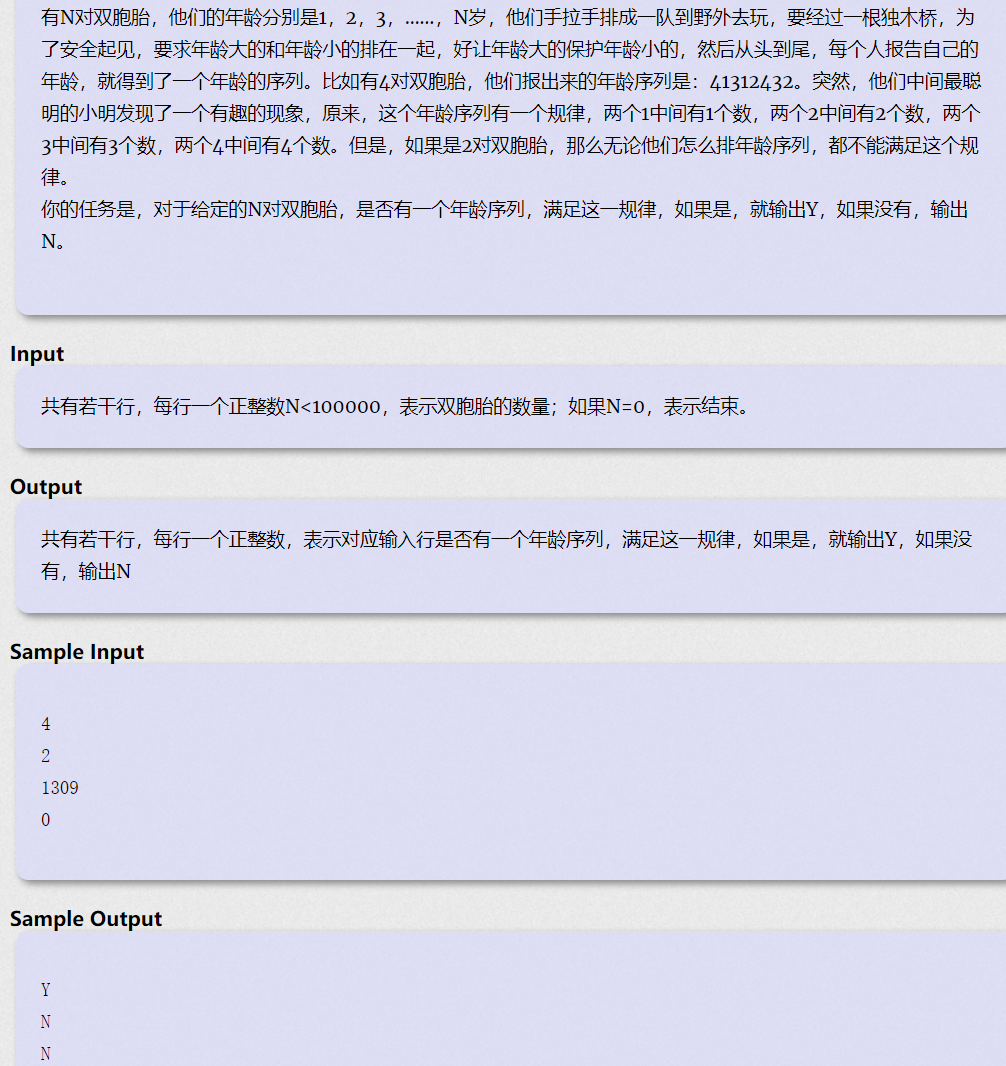Input

Output

Example
Input

3
4
1 7 6 5
5
1 2 3 4 5
2
0 -4

Output

2
0
3

Note

# T - Puzzle From the Future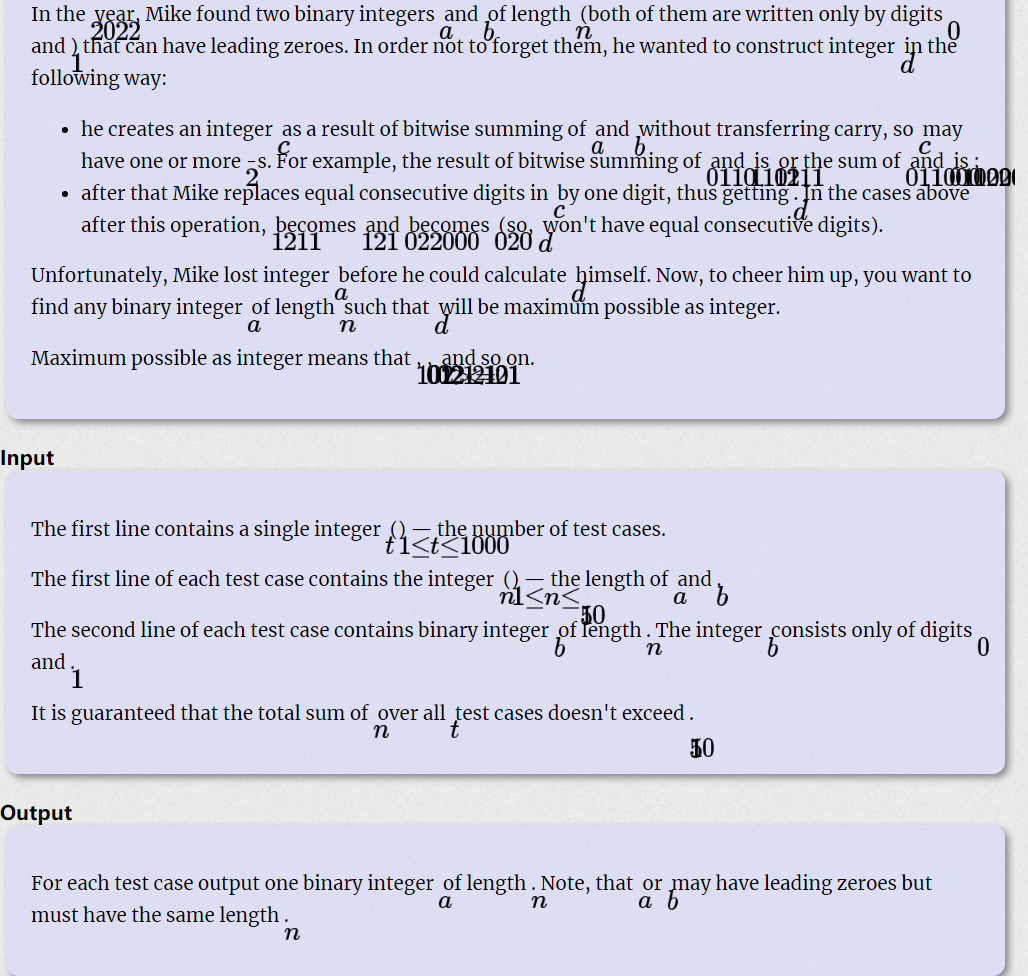Example
Input
5
1
0
3
011
3
110
6
111000
6
001011
Output
1
110
100
101101
101110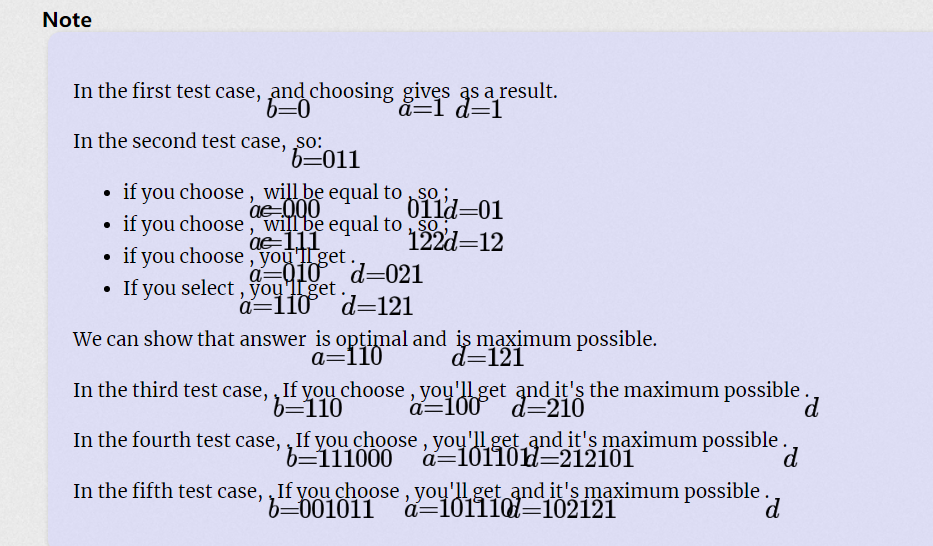暂无相关的数据...